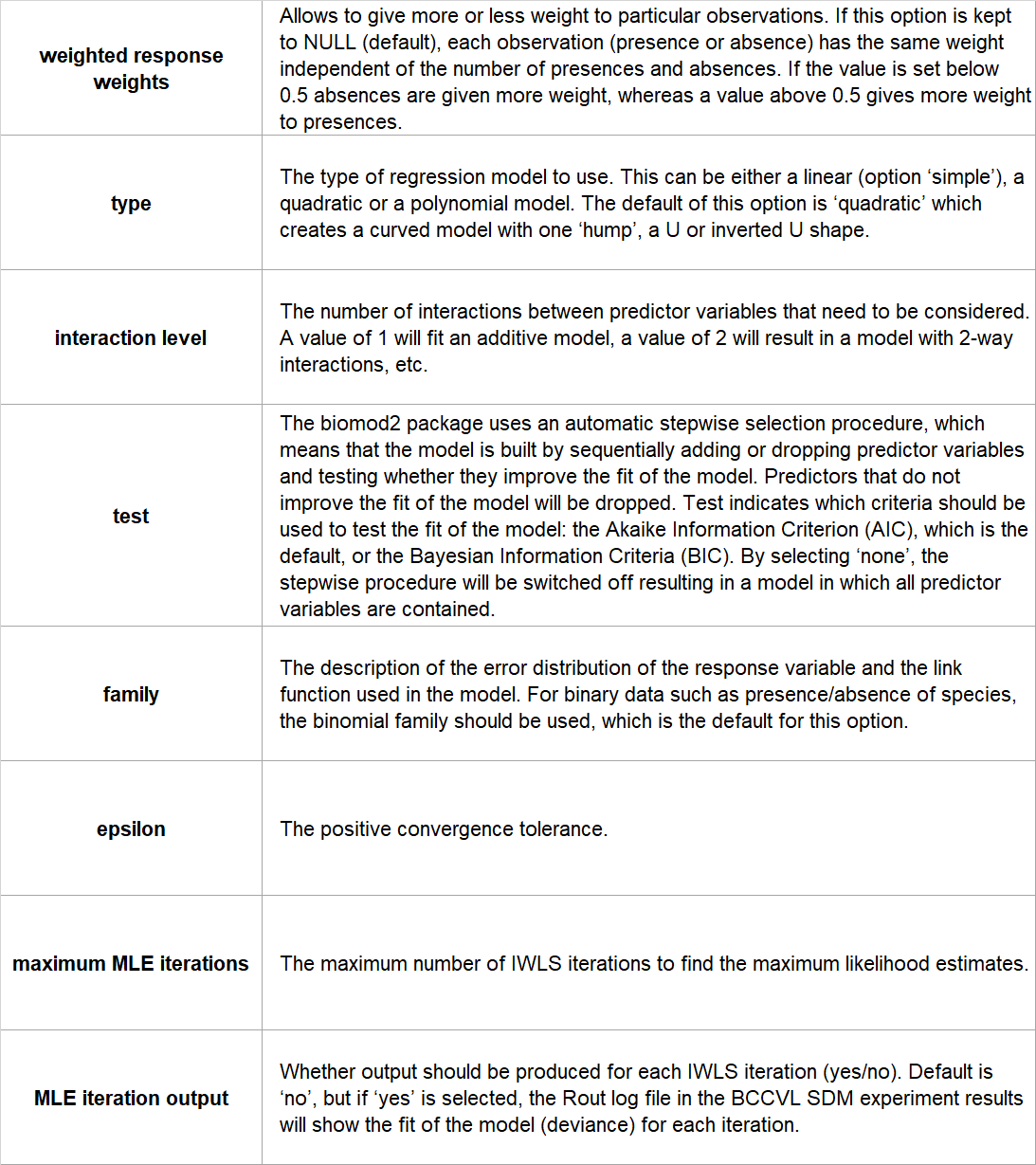### Introduction

Generalized Linear Models (GLM) are an extension of ‘simple’ linear regression models, which predict the response variable as a function of multiple predictor variables. Linear regression models work on a few assumptions, such as the assumption that we can use a straight line to describe the relationship between the response and the predictor variables. This implies that a constant change in a predictor leads to a constant change in the response variable. This assumption is often violated in ecological data, and therefore these models are extended into GLMs to be able to deal with non-normal distributed data.

GLMs find the equation that best predicts the occurrence of a species for the values of the environmental variables. The model has three important components:

1. The probability distribution of the response variable.
2. The linear predictor (LP): a combination of all predictor variables, which represents an overall score for the environmental suitability.
3. The link function: this describes how the mean of the response depends on the linear predictor.

Thus the predictors are linear, but the relationship between the response and the predictors is not linear, and the link function provides a transformation of the response so that the transformed response is linearly related to the predictors.

A GLM with binomial data, such as the presence/absence of a species, is commonly called “logistic regression”. In this case, the link function is a logit function, which is the log of the odds ratio (probability of presence/probability of absence).The coefficient of a predictor variable (the number that is used to multiply a variable) in a logistic regression model can be easily interpreted, as in the following hypothetical example. If a predictor, such as average annual temperature, has a positive coefficient of 0.3 in an estimated model of the occurrence of a species, this implies that a one unit increase in temperature results in an increase of exp(0.3) = 1.35 (the log-odds ratio), or 35%, in the probability of species presence.

The estimation of the values of the variable coefficients is obtained by maximum likelihood estimation (MLE), which maximizes the "agreement" of the predicted species occurrences with the observed data. In other words, MLE finds the values of the coefficients that result in a model under which you would be most likely to get the observed results. Most GLM models, including the GLM provided in BCCVL, use the iteratively reweighted least squares (IWLS) method for MLE.

• The response variable can have any form of exponential distribution type
• Able to deal with categorical predictors
• Relatively easy to interpret and allows a clear understanding of how each of the predictors are influencing the outcome
• Less susceptible to overfitting than for example CTA or MARS algorithms

### Limitations

• Needs relatively large datasets. The more predictor variables, the larger the sample size (N) required. As a rule of thumb, the number of predictor variables should be less than N/10.
• Sensitive to outliers

### Assumptions

No assumptions are made about the distributions of the environmental variables. However, they should not be highly correlated with one another because this could cause problems with the estimation.

Yes.

### Configuration options

BCCVL uses the ‘glm’ function in the ‘stats’ package, implemented in biomod2. The user can set the following configuration options:### References

• Elith J, Graham CH, Anderson RP et al. (2006) Novel methods improve prediction of species’ distributions from occurrence data. Ecography, 29(2), 129-151.
• Franklin J (2010) Mapping species distributions: spatial inference and prediction. Cambridge University Press.
• Guisan A, Edwards TC, Hastie T (2002) Generalized linear and generalized additive models in studies of species distributions: setting the scene. Ecological modelling, 157(2), 89-100.
• Hastie T, Tibshirani R, Friedman J (2009) The elements of statistical learning: data mining, inference and prediction. 2nd edition, Springer.